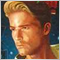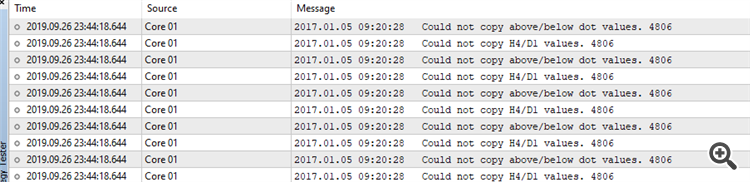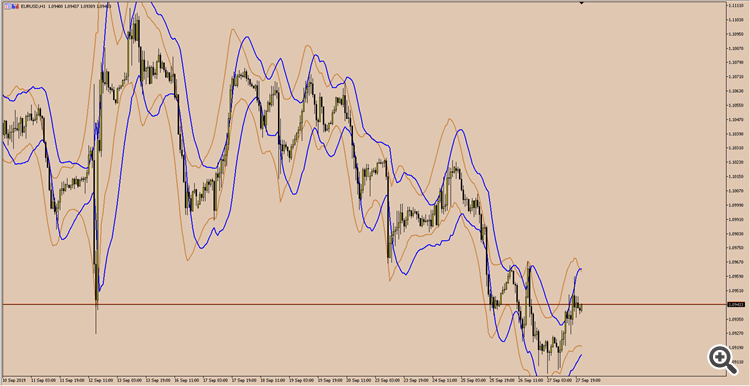Code not working for mt5, no problems with mt4, strange results43

I have this piece of code in an indi in mt4 which works fine:

for (int x = periods; x >= 0; x--) {
for (int i = pr + x; i > x; i--) {
volful[x] += (double)iVolume(_Symbol, PERIOD_CURRENT, i);
Print(x + " "  + volful[x] + " " + iVolume(_Symbol, PERIOD_CURRENT, i));
}
}

However when I try to use it with mt5 I get some strange results ending in bad numbers (even negative) & program break (divide by zero).

This is just supposed to sum the volume of i consecutive candles every candle, so number should be positive (& an integer - long) in any case. iVolume number comes correct, the adding part is failing though.

Here are the results with mt5:

Sometimes it works, most of the time not. Any idea?19843

volful[x] += (double)iVolume(_Symbol, PERIOD_CURRENT, i);
What did you initialize volful[x] to?43

It wasn't initialized, thought it would be like mt4 to 0 after array resize. It seems to be working now with ArrayInitialize.

Thank you.561

Always initialize, not only with arrays. Will save you a bunch of headache.43

Still facing issues with the dreaded 4806 but this time with the EA pointing to previous indi.

//-------------------------------------------------------------------------
int OnInit()
{
arrows = iCustom(_Symbol, PERIOD_CURRENT, "RibbonWaveCombo - new", 20, 50, 100, 200, MODE_LWMA, 0);
Sleep(1000);
return(INIT_SUCCEEDED);
}
//-------------------------------------------------------------------------
void OnTick()
{
if(OrdersTotal()>0) return;

ArrayResize(uparr, 3); ArrayResize(dnarr, 3); ArrayResize(buparr, 3); ArrayResize(bdnarr, 3);
ArrayInitialize(uparr, 0); ArrayInitialize(dnarr, 0); ArrayInitialize(buparr, 0); ArrayInitialize(bdnarr, 0);
ArraySetAsSeries(uparr, true); ArraySetAsSeries(dnarr, true); ArraySetAsSeries(buparr, true); ArraySetAsSeries(bdnarr, true);

ArrayResize(upwave, 3); ArrayResize(dnwave, 3);
ArrayInitialize(upwave, 0); ArrayInitialize(dnwave, 0);
ArraySetAsSeries(upwave, true); ArraySetAsSeries(dnwave, true);

ArrayResize(h4wave, 3); ArrayResize(d1wave, 3);
ArrayInitialize(h4wave, 0); ArrayInitialize(d1wave, 0);
ArraySetAsSeries(h4wave, true); ArraySetAsSeries(d1wave, true);

ArrayResize(clos, 3);
ArrayInitialize(clos, 0);
ArraySetAsSeries(clos, true);

if (CopyBuffer(arrows, 37, 0, 3, uparr) <= 0 ||
CopyBuffer(arrows, 38, 0, 3, dnarr) <= 0 ||
CopyBuffer(arrows, 39, 0, 3, buparr) <= 0 ||
CopyBuffer(arrows, 40, 0, 3, bdnarr) <= 0)
Print("Could not copy above/below dot values. ", GetLastError());
if (CopyBuffer(arrows, 5, 0, 3, upwave) <= 0 ||
CopyBuffer(arrows, 6, 0, 3, dnwave) <= 0)
Print("Could not copy high/low wave values. ", GetLastError());
if (CopyBuffer(arrows, 36, 0, 1, h4wave) <= 0 ||
CopyBuffer(arrows, 35, 0, 1, d1wave) <= 0)
Print("Could not copy H4/D1 values. ", GetLastError());

if (CopyClose(_Symbol, PERIOD_CURRENT, 0, 3, clos) <= 0)
Print("Could not copy close values. ", GetLastError());

if (clos < dnwave && buparr != EMPTY_VALUE && bdnarr != EMPTY_VALUE && uparr != EMPTY_VALUE && Total(1) == 0 && h4wave == 1 && d1wave == 1) { Trade(1, Lots, SL, TP); }
if (clos > upwave && bdnarr != EMPTY_VALUE && buparr != EMPTY_VALUE && dnarr != EMPTY_VALUE && Total(-1) == 0 && h4wave == 0 && d1wave == 0) { Trade(-1, Lots, SL, TP); }
/*
s = "N/A";
if (Close(1) > ema && he > he && r > LL && r <= LL && Total(1) == 0 && Time(0) > loc) { Trade(1, Lots, SL, TP); s = "Buy"; }
if (Close(1) < ema && he < he && r < UL && r >= UL && Total(-1) == 0 && Time(0) > loc) { Trade(-1, Lots, SL, TP); s = "Sell"; }
*/
}

I tried with both 0 delay, 1k delay, 10k delay, still get 4806 for parts of the indi:Goes on like that for the whole 2017.

It was working previously by invoking one indi which was then invoking another via icustom but it was too slow. Combined everything under one indi and it fails while the indi works better & faster when placed on a chart.

The corresponding parts in the indi are simple:

SetIndexBuffer(5, ma45h, INDICATOR_DATA);
SetIndexBuffer(6, ma45l, INDICATOR_DATA);

SetIndexBuffer(35, D1, INDICATOR_DATA);
SetIndexBuffer(36, H4, INDICATOR_DATA);
//RibbonWaveCombo-data
SetIndexBuffer(37, above, INDICATOR_DATA);
SetIndexBuffer(38, below, INDICATOR_DATA);
SetIndexBuffer(39, babove, INDICATOR_DATA);
SetIndexBuffer(40, bbelow, INDICATOR_DATA);

The only change I added on the indi was a 1ms delay via ontimer as it wasn't loading the higher tf ma's properly (h4, d1 & w1). Tried with ArrayResize/Initialize/SetAsSeries part also under OnInit but still no go.39023

sakisf112:

Still facing issues with the dreaded 4806 but this time with the EA pointing to previous indi.

I tried with both 0 delay, 1k delay, 10k delay, still get 4806 for parts of the indi:

Goes on like that for the whole 2017.

It was working previously by invoking one indi which was then invoking another via icustom but it was too slow. Combined everything under one indi and it fails while the indi works better & faster when placed on a chart.

The corresponding parts in the indi are simple:

The only change I added on the indi was a 1ms delay via ontimer as it wasn't loading the higher tf ma's properly (h4, d1 & w1). Tried with ArrayResize/Initialize/SetAsSeries part also under OnInit but still no go.

The problem is your indicator, fix it.43

Alain Verleyen:
The problem is your indicator, fix it.

Yeah, I guess so. I did some profiling and it seems that the problem is imaonarray. Translation of imaonarray from mql4 to mql5 is way too slow, mt4 never had a problem handling the same indi & ea without errors but I need faster optimization.43

Any idea why ExponentialMAOnBuffer won't produce the same values as iMAOnArray? MT5 & MT4 show identical values with imaonarray but it's stupid slow on mt5. Isn't ExponentialMAOnBuffer supposed to do the same thing?

for (int x = waveperiods; x >= 0; x--) {
dippe[x] = iHigh(_Symbol, PERIOD_CURRENT, x + 1) + atrix[x + 1] + cvolp[x] * atrix[x + 1];
kippe[x] = iLow(_Symbol, PERIOD_CURRENT, x + 1) - atrix[x + 1] - cvoln[x] * atrix[x + 1];
}
}

{ // YELLOW LINES
double MA1[]; ArrayResize(MA1, rates_total + 1);
ArrayInitialize(MA1, 0);
double MA2[]; ArrayResize(MA2, rates_total + 1);
ArrayInitialize(MA2, 0);
double MA3[]; ArrayResize(MA3, rates_total + 1);
ArrayInitialize(MA3, 0);
double MA4[]; ArrayResize(MA4, rates_total + 1);
ArrayInitialize(MA4, 0);

//ArraySetAsSeries(MA1, true);
//ArraySetAsSeries(MA3, true);
//ArraySetAsSeries(MA2, true);
//ArraySetAsSeries(MA4, true);

ExponentialMAOnBuffer(rates_total, prev_calculated, 0, Sampling_Period, dippe, MA1);
ExponentialMAOnBuffer(rates_total, prev_calculated, 0, Sampling_Period, kippe, MA3);

ExponentialMAOnBuffer(rates_total, prev_calculated, 0, wpr, MA1, MA2);
ExponentialMAOnBuffer(rates_total, prev_calculated, 0, wpr, MA3, MA4);

/*
for (int i = waveperiods; i >= 0; i--) {
MA1[i] = iMAOnArrayMQL4(dippe, 0, Sampling_Period, 0, MODE_EMA, i);
MA3[i] = iMAOnArrayMQL4(kippe, 0, Sampling_Period, 0, MODE_EMA, i);
}

for (int i = waveperiods; i >= 0; i--) {
MA2[i] = iMAOnArrayMQL4(MA1, 0, wpr, 0, MODE_EMA, i);
MA4[i] = iMAOnArrayMQL4(MA3, 0, wpr, 0, MODE_EMA, i);
}
*/
Blue is the correct one with iMAonArray